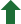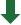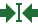### The basics of Faust, Formally!

We have already seen quite a bit about Faust, can we build a formal model in Coq? The answer of course, is yes.

#### Representing Faust Programs inside Coq

The standard technique to start with program representation is to capture Faust's syntax as a datatype.

Coq's datatypes are so-called inductively-defined datatypes, meaning that an element of a type is usually built out of several cases; we use a simplified version of Faust for educational purposes:

#### Making sense Faust programs!

We have built a representation of Faust programs, but now we'll like to capture formally their effect on sound too.

Thus, we will build an interpretation function, which basically will take a Faust program and return a Coq's function from signals to singal:

$$⟦ f : i ↝ o ⟧ : S^i \to S^o$$

Now the question is what should our signal type $S$, be? We have assumed the basic data type of sampled to be some algebraic field, given that Faust operates at a 1-sample rate, it is convenient to encode signals as list of length $n$, where $n$ is the output produced at the $n^{th}$ step:

Then, the interpreation function only has to run every particular singal processor for $n$ steps:

#### A sample logic for Faust programs

We can check that the semantics meet the expected properties. And indeed, we can even use more general theorems to check properites about Faust. A common technique in formal verification is to use a program logic, which is then used to reason about programs. Our sample-level logic for Faust looks as follows:

#### Soundess and interpretation of a judgment

We need to prove our logic sound, then we can profit from it!

#### Checking stability of our simple smooth filter.

We can use this technique to prove out little filter stable, that is:

$$\forall x~c, x \in [-1,1] \to c \in [0,1] \to ⟦ \mathit{smooth(c)} ⟧(x) \in [-1,1]$$

which unfolding the signal representation amounts to:

$$\forall x~c~n, x(n) \in [-1,1] \to c \in [0,1] \to ⟦ \mathit{smooth(c)} ⟧(x)(n) \in [-1,1]$$

The proof is straightforward using the logic, using some interval aritmetic in Coq.